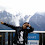## Monday 3 September 2012

### CBSE Class 9 - Maths - Ch 7 Triangles (MCQs)

Trianglescredits:openclipart

Q1: In Δ ABC, AB = AC and ∠B = 50°. Then ∠C is equal to

(a) 40°
(b) 50°
(c) 80°
(d) 130°

Q2(CBSE 2010): In ΔABC, ∠C = ∠A and BC = 6 cm and AC = 5 cm. Then the length of AB is:

(a) 6 cm
(b) 5 cm
(c) 3 cm
(d) 2.5 cm

Q3: If one angle of a triangle is equal to the sum of other two angles, then the triangle is

(a) an isosceles triangle
(b) an obtuse triangle
(c) an equilateral triangle
(d) a right triangle

Q4(CBSE 2011): If ΔABC, is right angled at B, then :

(a) AB = AC
(b) AC < AB
(c) AB = BC
(d) AC > AB

Q5(CBSE 2011): In ΔABC if AB = BC, then :

(a) ∠B > ∠C
(b) ∠A = ∠C
(c) ∠A = ∠B
(d) ∠A < ∠B

Q6(CBSE 2010):  In ΔPQR, ∠P = 60°, ∠Q = 50°. Which side of the triangle is the longest ?

(a) PQ
(b) QR
(c) PR
(d) none

Q7(CBSE 2011): P is a point on side BC of  Δ ABC, such that AP bisects ∠BAC, then :

(a) BP = CP
(b) BA > BP
(c) BP > BA
(d) CP < CA

Q8(CBSE 2011):  In the give figure q-8, AD is the median, then ∠BAD  is:
(a) 55°
(b) 50°
(c) 100°
(d) 40°

Q9(CBSE 2010):  If ΔABC ≅ ΔDEF by SSS congruence rule then :

(a) AB = EF, BC = FD, CA = DE
(b) AB = FD, BC = DE, CA = EF
(c) AB = DE, BC = EF, CA = FD
(d) AB = DE, BC = EF, ∠C = ∠F

Q10(CBSE 2011): If  Δ ABC is a right angled at B, then

(a) AB = AC
(b) AC < AB
(c) AB = AC
(d) AC > AB

Q11: In a Δ ABC, ∠A = ∠C. If BC = 3 and  AC = 4 then the perimeter of the triangle is:

(a) 7
(b) 10
(c) 12
(d) 14

Q12: From the following which condition is not possible for the congruence of two triangles ?
(a) ASA
(b) AAS
(c) AAA
(d) SSS

Q13: In a right angled triangle, if one acute angle is half the other, then the smallest angle is:

(a) 15°
(b) 25°
(c) 30°
(d) 35°

1: (b) 50° [Hint: Δ ABC, AB = AC is an isosceles triangle, ∠B =∠C ]

2: (a) 6 cm [ Hint: Δ ABC, ∠C = ∠A, an isosceles triangle, BC = AB]

3: (d) a right triangle
[Hint: Δ ABC, ∠A = ∠B + ∠C, ∵ ∠A + ∠B + ∠C = 180° ⇒ 2∠A = 180° ⇒ ∠A = 90° ]

4: (d) AC > AB [ Hint: Δ ABC, ∠B = 90°, AC is hypotenuse]

5: (b) ∠A = ∠C [Hint: Δ ABC, AB = BC is an isosceles triangle, ∠A =∠C ]

6: (a) PQ [Hint: ∠R = 70° is the largest, the side opposite to the largest angle is the longest.]

7:  (b) BA > BP
[Hint: Consider the triangle as show in fig-q7,
Given, ∠BAP = ∠CAP = z (say)
∠APC is an exterior angle for ΔBAP
⇒ ∠APC = ∠ABP + ∠BAP
⇒ ∠APC > ∠BAP (greater angle has greater side opposite to it.)
⇒ BA > BP]

8: (b) 50°
[Hint:AB = AC ⇒ ∠ABD = ∠ACD = 40°. ⇒ ∠BAC = 100°
Δ ABD ≅  Δ ACD by SSS congruence

9: (c) AB = DE, BC = EF, CA = FD
[Hint: Remember CPCT for corresponding parts of congruent triangles.]

10: (d) AC > AB
[Hint:  ∠B = 90°, AC is hypotenuse the longest side. ]

11: (b) 10 [Hint:  ∠A = ∠C, ⇒AB = BC = 3.]

12: (c) AAA

13: (c) 30° [Hint: x + 2x + 90° = 180° ⇒ 3x = 90°  ⇒ x = 30° ]

.1.good selection of mcq's

2.3.4.5.In 7th question (d) can also be right

6.This comment has been removed by the author.

7.This helped me for my MCQ test

8.9.not so good but a little helpful very little

10.11.should put mcq's of all chapter it is very helpful

12.thank you this the best mcq's

13.These were too easy and simple I have done this MCQ questions so simply because I am so genius in maths and no one can losse me in math

14.very much improved me in my mcq questions. Very much helpful